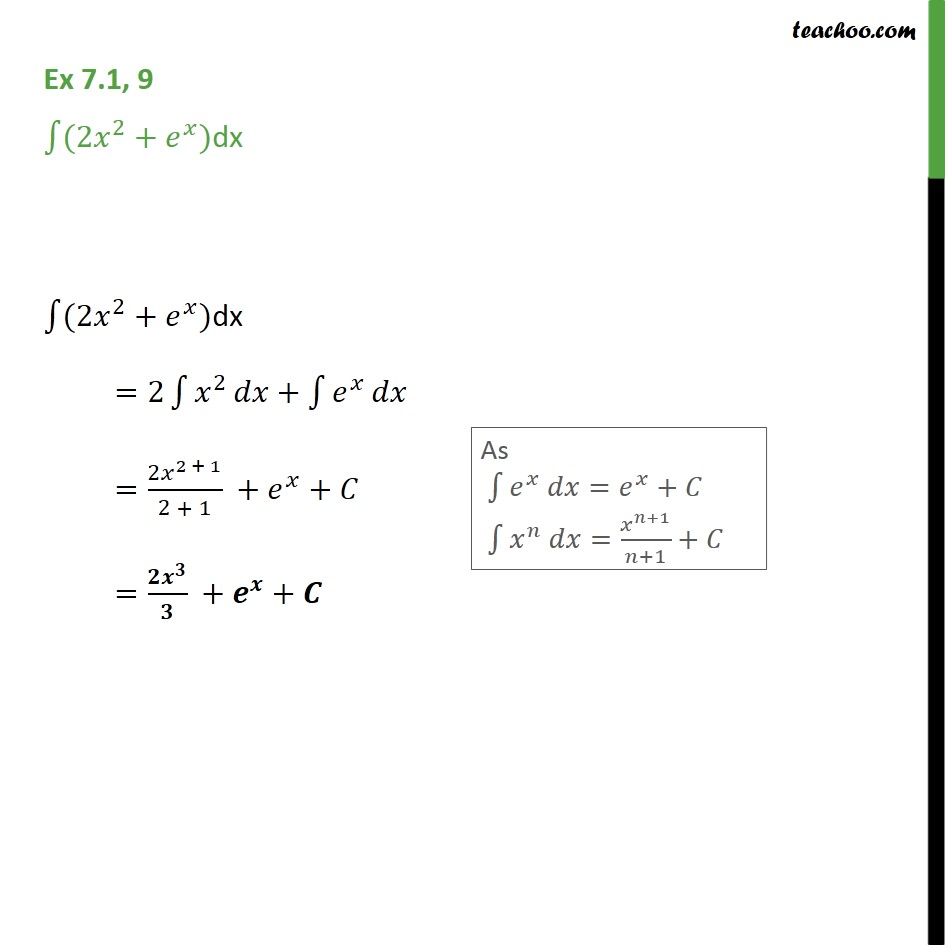1. Chapter 7 Class 12 Integrals (Term 2)
2. Serial order wise
3. Ex 7.1

Transcript

Ex 7.1, 9 - Chapter 7 Class 12 Integrals - NCERT Solution ∫ (2x^2 + e^x) dx ∫ (2x^2 + e^x) dx = 2∫ x^2 dx + ∫ e^x dx =〖2𝑥〗^(2 + 1)/(2 + 1) +𝑒^𝑥+𝐶 As ∫1▒〖𝑒^𝑥 𝑑𝑥=𝑒^𝑥+𝐶〗 ∫1▒〖𝑥^𝑛 𝑑𝑥=𝑥^(𝑛+1)/(𝑛+1)+𝐶〗 =(𝟐𝒙^𝟑)/𝟑 +𝒆^𝒙+𝑪

Ex 7.1